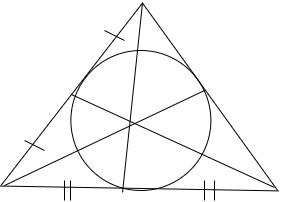# What is a nine-point circle?

The approach for geometry can be observed from ancient times in their constructions from the use of various shapes in a very specific way. The term is originally derived from Greek words ‘geo’ and ‘materia’ which means earth and measurement respectively. Let’s start by understanding the definition of geometry,

### Geometry

Geometry is a part of the development of the modern world. Modernized systems highly depend on geometry as it is used for designing, constructional works, architecture in choice of material for construction, and many more. It is also applicable in the technical background for computing various designs, manufacturing, creating blueprints, programming, etc. Geometry is a branch of mathematics that deals with the study of shapes and their properties. The given article is a study about the nine-point circle, its properties, and step by step method to construct a nine-point circle.

### What is a nine-point circle?

Nine point circle is a circle that is constructed in any given triangle. It is also known as the Feuerbach’s circle named after its discoverer. The circle constructed passes through 3 concyclic points from the triangle. The center of it lies at the nine-point center of the triangle, and the radius of the nine-point circle is exactly half of the circumradius of the triangle that is N = 1/2R. The nine-point circle was discovered by Feuerbach in 1820.

The nine points the circle passed through from the triangle are:

• The midpoint of the three sides
• The foot of each altitude.
• And midpoint of the line segment from each vertex of the triangle to its orthocenter.Construction of nine-point circle

To draw a nine-point circle, follow certain steps,

• Step1: Draw a triangle on a plane surface.
• Step 2: Mark the midpoint of the sides of the triangle.
• Step 3: Draw an altitude to the opposite side from each vertex and mark the points.
• Step 4: Now, mark the common point at which the altitudes intersect.

### Sample Problems

Question 1: What is the radius of the point circle?

The radius of point circle is the distance from the circles center to a point on the circle.

Question 2: List the circle formulas.

• Diameter of circle

D = 2r

• circumference of circle

C = 2πr

• Area of circle

A = πr2

Question 3: Give the equation of the point circle?

The equation for a point circle is x2 + y2 + 2gx + 2fy + c = 0.

Question 4: What is the radius of the nine-point circle?

The radius of nine point circle is exactly half of the circumradius of triangle which is given by N = 1/2R.

Question 5: What are the nine points the circle passed through from the triangle?